Learning Library

Input/Output Tables

Challenge your math whiz to review his basic math operations by discovering the secret function that changes the "input" to the "output".

Click on a worksheet in the set below to see more info or download the PDF.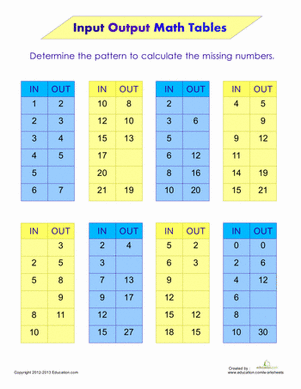Input Output Math Tables

Find the hidden rule in these puzzling math tables! Compare your "in" number to the corresponding "out" number to find the pattern in each table.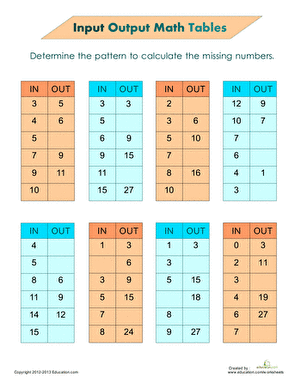Function Tables

Compare your "input" number to the corresponding "output" number to find the pattern in each table and fill in the missing numbers.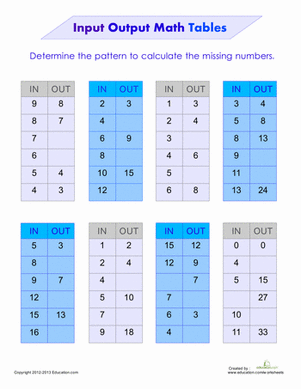Function Tables: Input Output

Find the rule that links the input and output numbers in this chart! It could be addition, subtraction or multiplication; it's up to you to find the connection.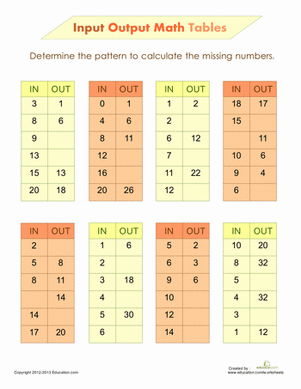Input Output Math

Use a little bit of logic to find out the rule that links "input" and "output" in these math puzzles.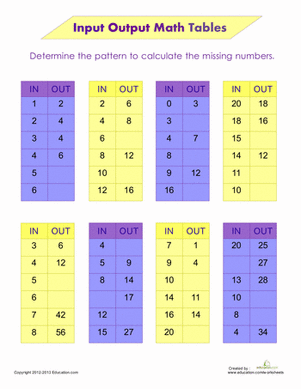Input and Output Tables

Find the mathematical rule in these math tables. Compare the input and output numbers to find the hidden pattern in each table.

Want to download this whole set as a single PDF?

Create new collection

0

New Collection>

0 items

What could we do to improve Education.com?

Please note: Use the Contact Us link at the bottom of our website for account-specific questions or issues.

What would make you love Education.com?